# EMT 1143 INTRODUCTION TO ELECTRIC CIRCUITS Chapter 2

• Slides: 31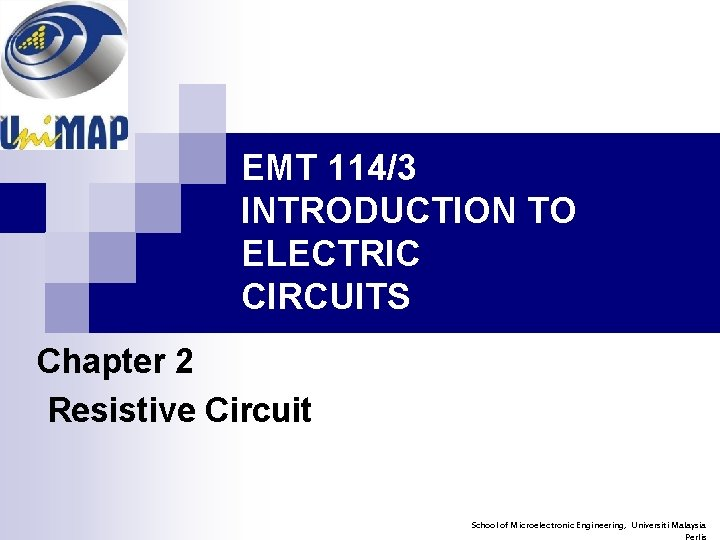EMT 114/3 INTRODUCTION TO ELECTRIC CIRCUITS Chapter 2 Resistive Circuit School of Microelectronic Engineering, Universiti Malaysia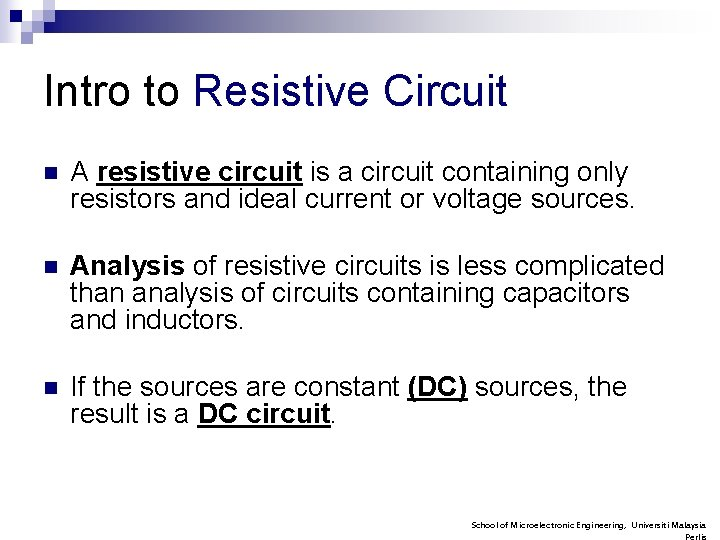Intro to Resistive Circuit n A resistive circuit is a circuit containing only resistors and ideal current or voltage sources. n Analysis of resistive circuits is less complicated than analysis of circuits containing capacitors and inductors. n If the sources are constant (DC) sources, the result is a DC circuit. School of Microelectronic Engineering, Universiti MalaysiaOutline 1. Series and Parallel Resistor 2. Voltage Divider 3. Current Divider 4. Voltage and Current Measurements 5. Wheatstone Bridge 6. Delta-Wye Equivalent Circuits School of Microelectronic Engineering, Universiti Malaysia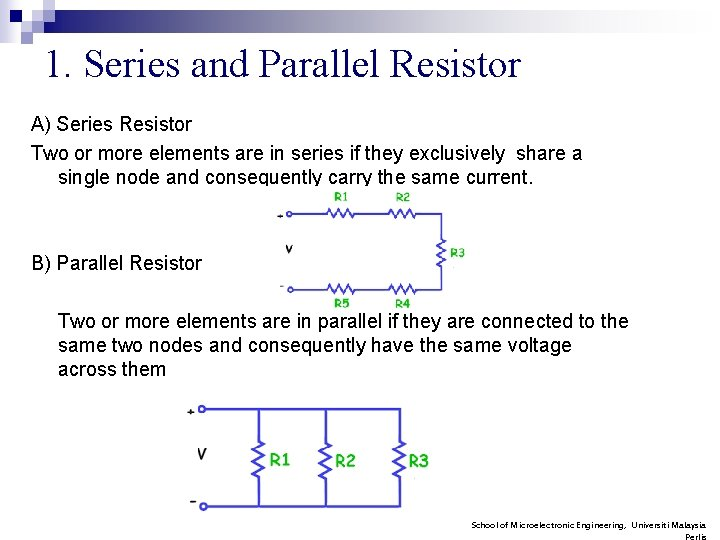1. Series and Parallel Resistor A) Series Resistor Two or more elements are in series if they exclusively share a single node and consequently carry the same current. B) Parallel Resistor Two or more elements are in parallel if they are connected to the same two nodes and consequently have the same voltage across them School of Microelectronic Engineering, Universiti Malaysia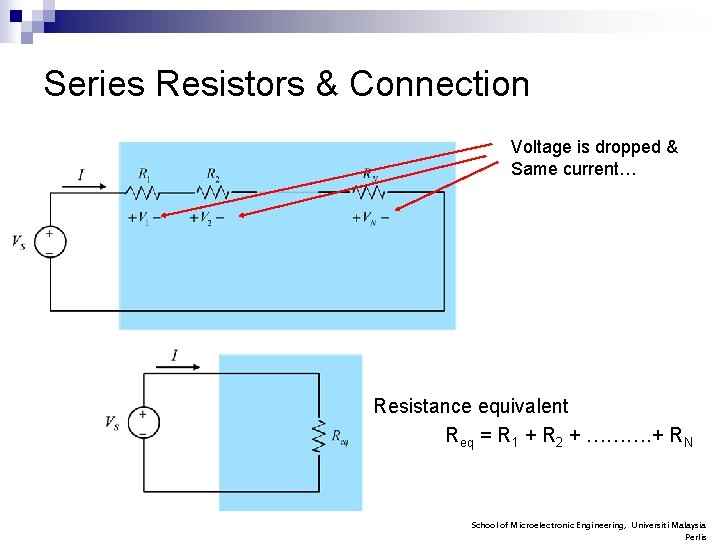Series Resistors & Connection Voltage is dropped & Same current… Resistance equivalent Req = R 1 + R 2 + ………. + RN School of Microelectronic Engineering, Universiti Malaysia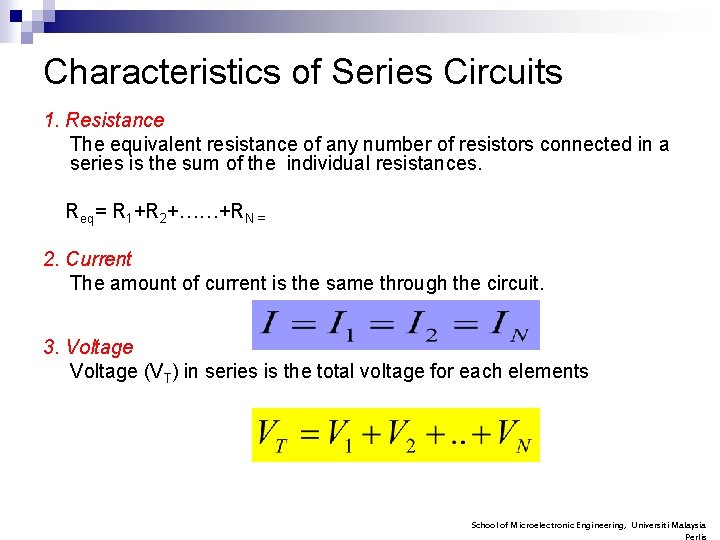Characteristics of Series Circuits 1. Resistance The equivalent resistance of any number of resistors connected in a series is the sum of the individual resistances. Req= R 1+R 2+……+RN = 2. Current The amount of current is the same through the circuit. 3. Voltage (VT) in series is the total voltage for each elements School of Microelectronic Engineering, Universiti Malaysia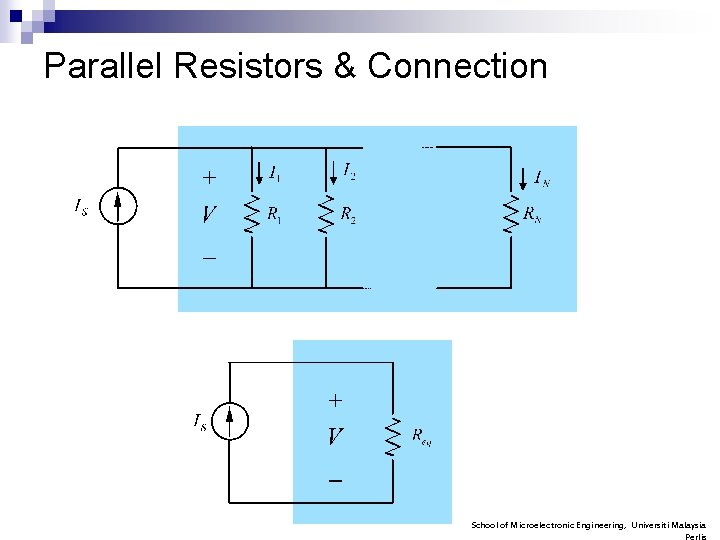Parallel Resistors & Connection School of Microelectronic Engineering, Universiti MalaysiaCharacteristics of parallel Circuits n Resistance The equivalent resistance is reciprocal of total resistance n Current in parallel circuits is the total current for each of the circuits elements n Voltage (VT) for parallel circuits is the same for all circuits elements School of Microelectronic Engineering, Universiti Malaysia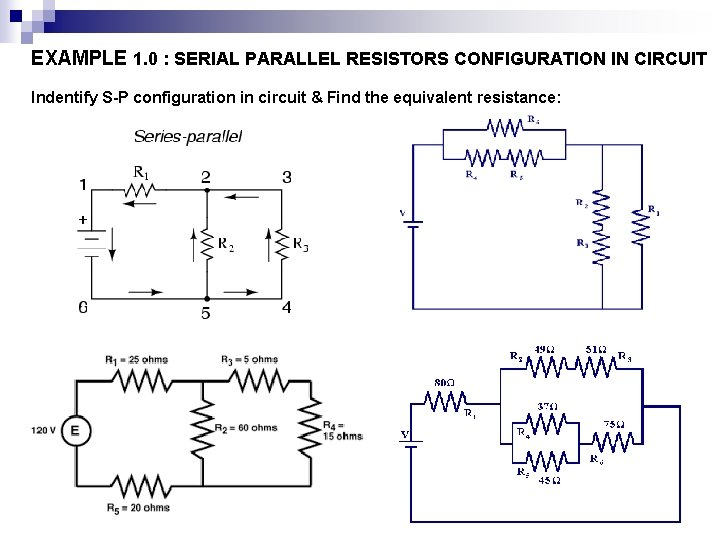EXAMPLE 1. 0 : SERIAL PARALLEL RESISTORS CONFIGURATION IN CIRCUIT Indentify S-P configuration in circuit & Find the equivalent resistance: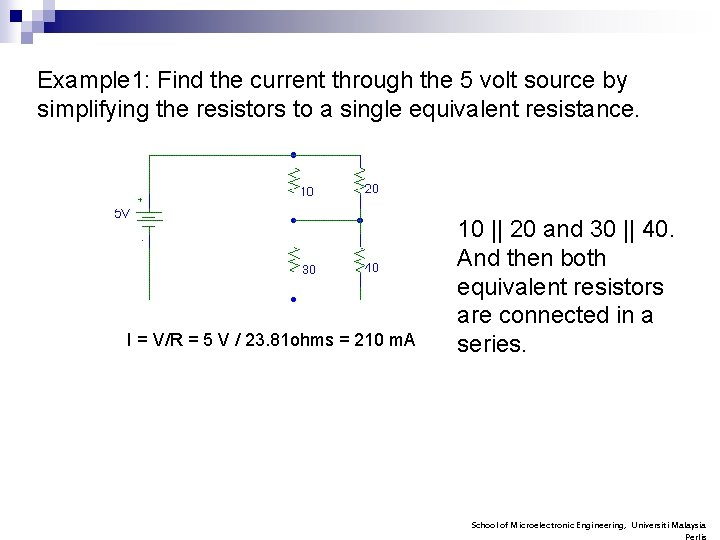Example 1: Find the current through the 5 volt source by simplifying the resistors to a single equivalent resistance. I = V/R = 5 V / 23. 81 ohms = 210 m. A 10 || 20 and 30 || 40. And then both equivalent resistors are connected in a series. School of Microelectronic Engineering, Universiti Malaysia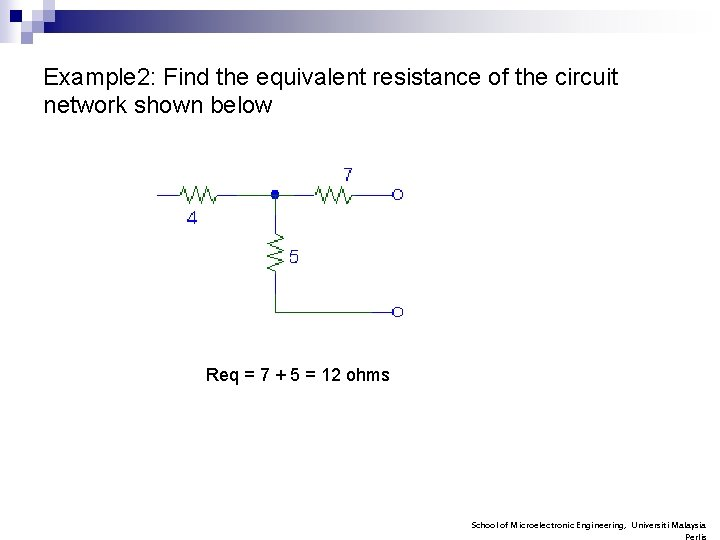Example 2: Find the equivalent resistance of the circuit network shown below Req = 7 + 5 = 12 ohms School of Microelectronic Engineering, Universiti Malaysia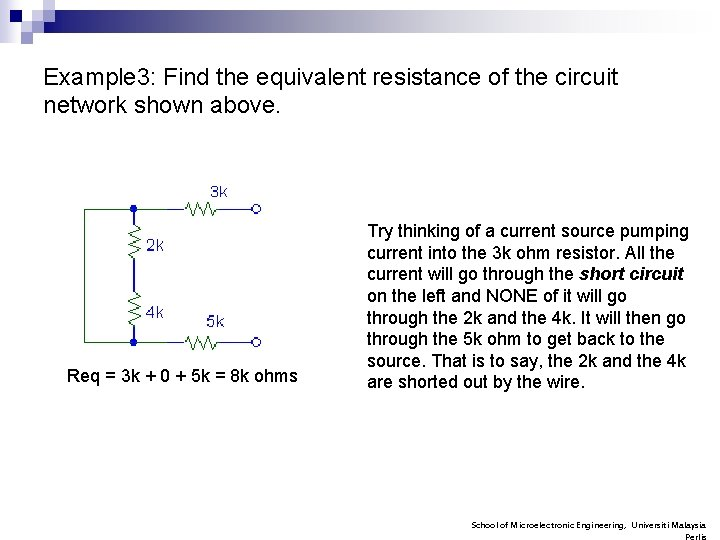Example 3: Find the equivalent resistance of the circuit network shown above. Req = 3 k + 0 + 5 k = 8 k ohms Try thinking of a current source pumping current into the 3 k ohm resistor. All the current will go through the short circuit on the left and NONE of it will go through the 2 k and the 4 k. It will then go through the 5 k ohm to get back to the source. That is to say, the 2 k and the 4 k are shorted out by the wire. School of Microelectronic Engineering, Universiti Malaysia2. Voltage Divider By Applying Ohms Law; V=IR So; V 1 = IR 1 V 2 = IR 2 V = V 1+ V 2 = I(R 1+R 2) ……eq 1 School of Microelectronic Engineering, Universiti MalaysiaCont. . n So to find voltage drop across R 2, I=V 2 / R 2 …………. In eq 1 V 2= (V/ R 1+R 2) x R 2 V 2 = In general, voltage drop across any resistor, or combination of resistors, in a series circuit is equal to the ratio of that resistance value to the total resistance, multiplied by the source voltage. School of Microelectronic Engineering, Universiti MalaysiaExample 4: Find the voltage drop across the resistors. School of Microelectronic Engineering, Universiti Malaysia3. Current Divider By Applying Ohms Law; V=IR V = I 1 R 1 and V= I 2 R 2, ----equation 1 Total current flow; V= IReq -----equation 2 Where, Req = (R 1 R 2/R 1+R 2) …how to get this Req? ? equation (2) in (1) IReq = I 1 R 1 Rearrange: School of Microelectronic Engineering, Universiti Malaysia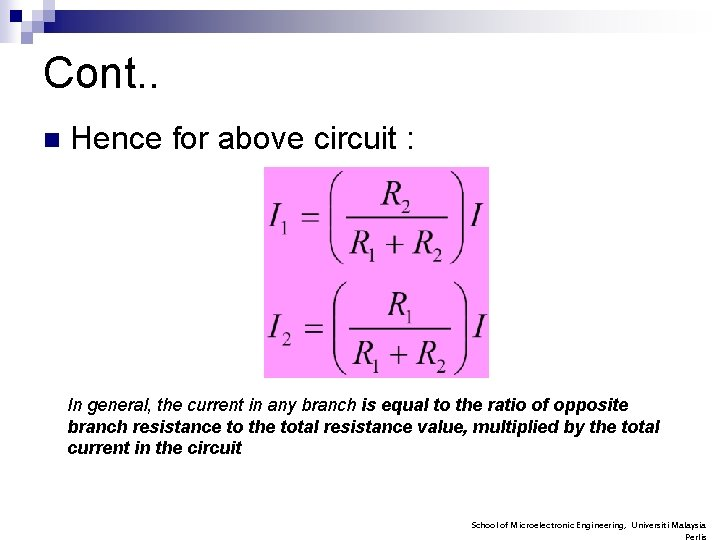Cont. . n Hence for above circuit : In general, the current in any branch is equal to the ratio of opposite branch resistance to the total resistance value, multiplied by the total current in the circuit School of Microelectronic Engineering, Universiti Malaysia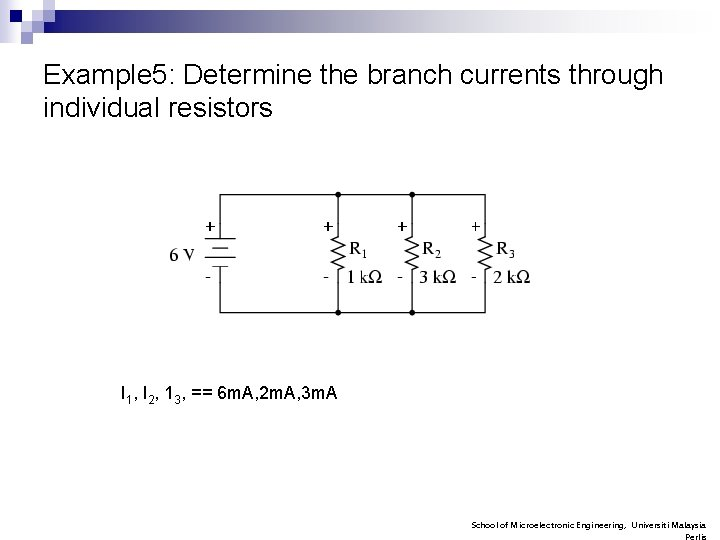Example 5: Determine the branch currents through individual resistors I 1, I 2, 13, == 6 m. A, 2 m. A, 3 m. A School of Microelectronic Engineering, Universiti Malaysia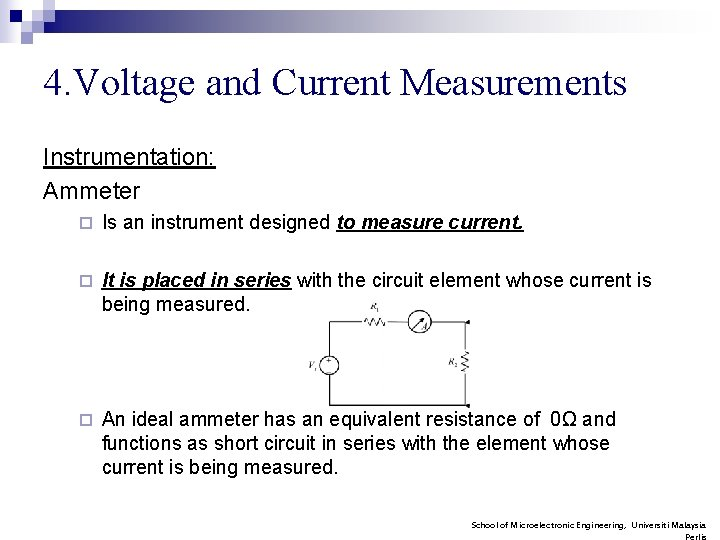4. Voltage and Current Measurements Instrumentation: Ammeter ¨ Is an instrument designed to measure current. ¨ It is placed in series with the circuit element whose current is being measured. ¨ An ideal ammeter has an equivalent resistance of 0Ω and functions as short circuit in series with the element whose current is being measured. School of Microelectronic Engineering, Universiti MalaysiaCont. . Voltmeter ¨ Is an instrument designed to measure potential different (voltage) between two point in the circuit. ¨ It is placed in parallel with the element whose voltage is being measured. ¨ An ideal voltmeter has infinite equivalent resistance and thus functions as an open circuit in parallel with the element whose voltage is being measured School of Microelectronic Engineering, Universiti MalaysiaCircuit configuration/placement Voltmeter connection with Ammeter and EXAMPLE School of Microelectronic Engineering, Universiti Malaysia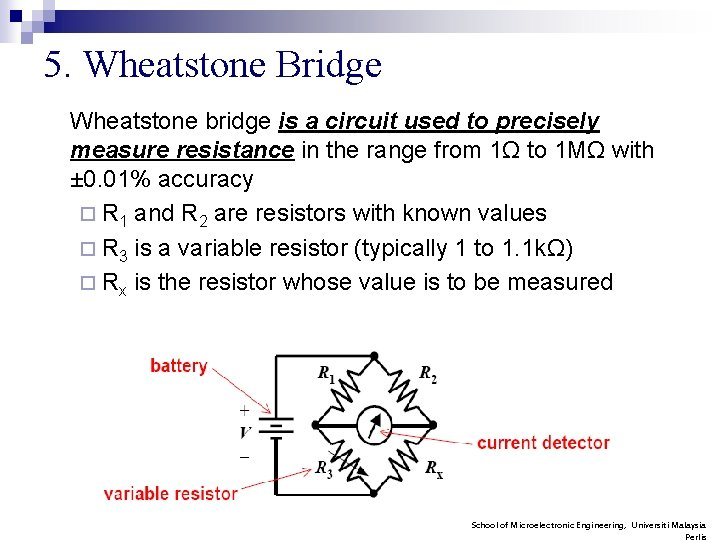5. Wheatstone Bridge Wheatstone bridge is a circuit used to precisely measure resistance in the range from 1Ω to 1 MΩ with ± 0. 01% accuracy ¨ R 1 and R 2 are resistors with known values ¨ R 3 is a variable resistor (typically 1 to 1. 1 kΩ) ¨ Rx is the resistor whose value is to be measured School of Microelectronic Engineering, Universiti Malaysia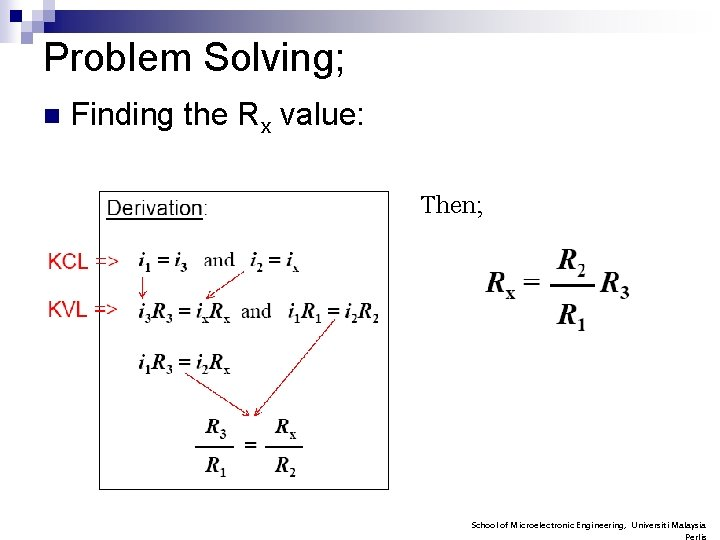Problem Solving; n Finding the Rx value: Then; School of Microelectronic Engineering, Universiti Malaysia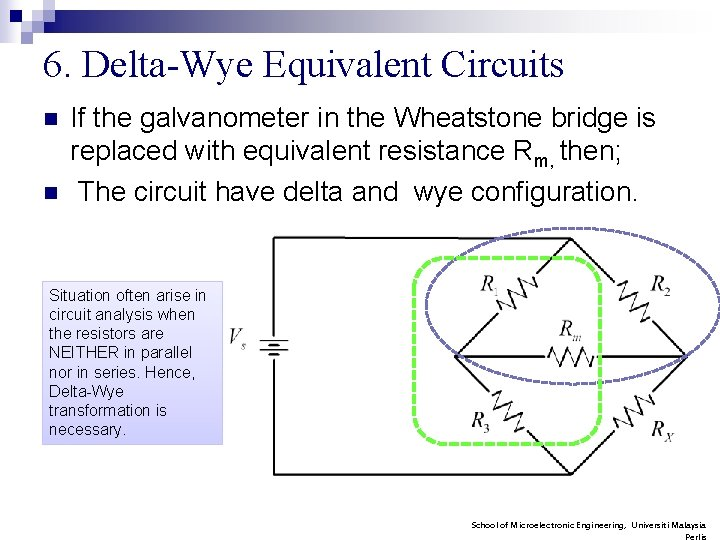6. Delta-Wye Equivalent Circuits n n If the galvanometer in the Wheatstone bridge is replaced with equivalent resistance Rm, then; The circuit have delta and wye configuration. Situation often arise in circuit analysis when the resistors are NEITHER in parallel nor in series. Hence, Delta-Wye transformation is necessary. School of Microelectronic Engineering, Universiti MalaysiaDelta (Δ) and Pi (π) n The resistor R 1, R 2 and Rm (or R 3, Rm and Rx) are referred as a Δ interconnection. It is also referred as a π interconnection because the Δ can be shaped into a π without disturbing the electrical equivalence of the two configurations. School of Microelectronic Engineering, Universiti MalaysiaWye(Y) and Tee(T) n The resistor R 1, R 3 and Rm (or R 2, Rm and Rx) are referred as a Wye (Y) interconnection because it can be look like letter Y. The Y configuration also referred as Tee (T) interconnection. School of Microelectronic Engineering, Universiti Malaysia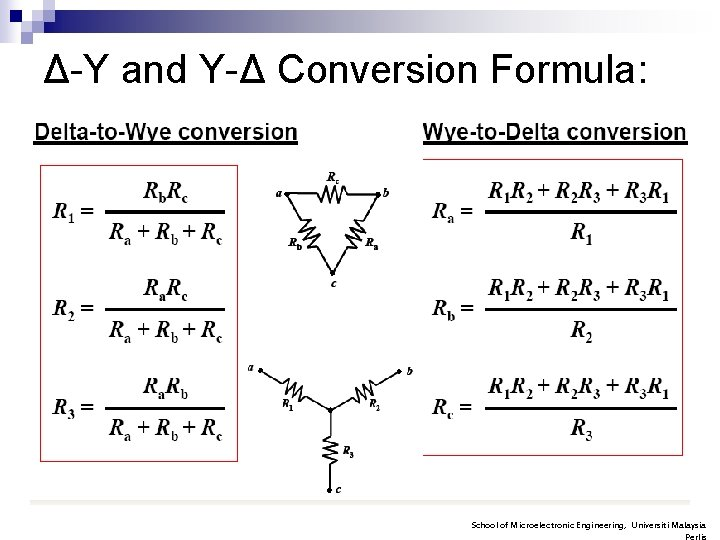Δ-Y and Y-Δ Conversion Formula: School of Microelectronic Engineering, Universiti MalaysiaExercise 1 Find the equivalent resistance for electrical network below a) b) n School of Microelectronic Engineering, Universiti MalaysiaExercise 2 n Given voltage, Vs= 12 V determine the current through the R 1 = 25 Ω and R 2. = 100Ω School of Microelectronic Engineering, Universiti Malaysia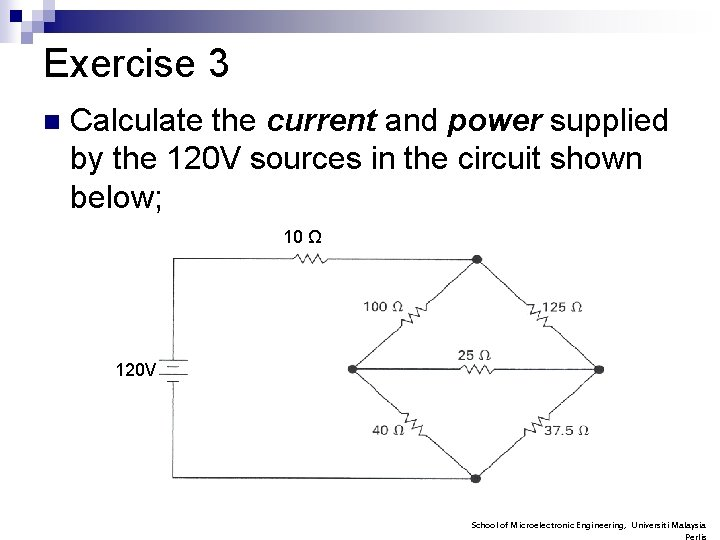Exercise 3 n Calculate the current and power supplied by the 120 V sources in the circuit shown below; 10 Ω 120 V School of Microelectronic Engineering, Universiti MalaysiaIlmu Keikhlasan Kecemerlangan School of Microelectronic Engineering, Universiti Malaysia